Courses

Test: Numbers from 21 to 50- 1

10 Questions MCQ Test Mathematics for Class 1 | Test: Numbers from 21 to 50- 1

Description
Attempt Test: Numbers from 21 to 50- 1 | 10 questions in 15 minutes | Mock test for Class 1 preparation | Free important questions MCQ to study Mathematics for Class 1 for Class 1 Exam | Download free PDF with solutions
QUESTION: 1

Ravi and Astha took a maths quiz. Ravi scored 6 marks out of 10. Astha scored more marks than Ravi. How many marks do you think Astha scored?

Solution:
• 9 is greater than 6.
• Astha scored more marks than Ravi.
• Ravi scored 6 marks and Astha would have scored 9 marks.
QUESTION: 2

Which of the following is correct?

Solution:

3 is smaller than 4 or 3 < 4

QUESTION: 3

Which of the following is correct?

Solution:

10 is greater than 9 or 10 > 9

QUESTION: 4

Which number is greater than 6?

Solution:

9 is greater than 6.

QUESTION: 5

Which sign shows that a number is equal to the other number?

Solution:

‘=’, sign shows the equal to sign.

QUESTION: 6

Which of the following baskets has 5 things in it?

Solution:

There are 5 fruits in the third basket.

QUESTION: 7

How many fish are there in this bowl?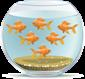Solution:

There are 6 fish in the fish bowl.

QUESTION: 8

birds are sitting on a branch.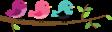After some time, 3 birds flew away. How many birds are now on the branch?Solution:

There are 0 birds left on the tree as all the 3 birds flew away.

QUESTION: 9

Choose the option that shows seven beads.

Solution: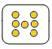QUESTION: 10

Count the number of bikes. Choose the correct option.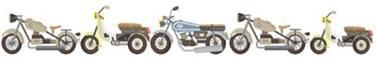Solution:

There are 5 bikes in all.Use Code STAYHOME200 and get INR 200 additional OFF Use Coupon Code

Track your progress, build streaks, highlight & save important lessons and more!

Similar Content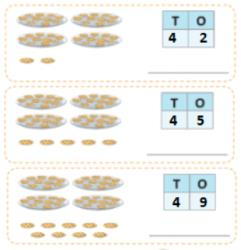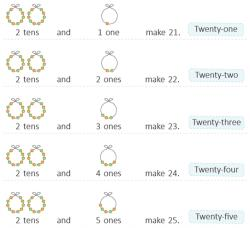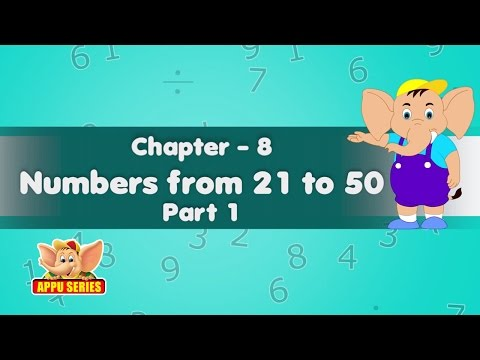Related tests